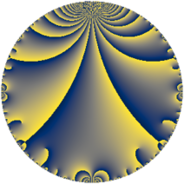# Properties

 Label 7.4.aLevel $7$ Weight $4$ Character orbit 7.a Rep. character $\chi_{7}(1,\cdot)$ Character field $\Q$ Dimension $1$ Newform subspaces $1$ Sturm bound $2$ Trace bound $0$

# Learn more about

## Defining parameters

 Level: $$N$$ $$=$$ $$7$$ Weight: $$k$$ $$=$$ $$4$$ Character orbit: $$[\chi]$$ $$=$$ 7.a (trivial) Character field: $$\Q$$ Newform subspaces: $$1$$ Sturm bound: $$2$$ Trace bound: $$0$$

## Dimensions

The following table gives the dimensions of various subspaces of $$M_{4}(\Gamma_0(7))$$.

Total New Old
Modular forms 3 1 2
Cusp forms 1 1 0
Eisenstein series 2 0 2

The following table gives the dimensions of the cuspidal new subspaces with specified eigenvalues for the Atkin-Lehner operators and the Fricke involution.

$$7$$Dim.
$$+$$$$1$$

## Trace form

 $$q - q^{2} - 2q^{3} - 7q^{4} + 16q^{5} + 2q^{6} - 7q^{7} + 15q^{8} - 23q^{9} + O(q^{10})$$ $$q - q^{2} - 2q^{3} - 7q^{4} + 16q^{5} + 2q^{6} - 7q^{7} + 15q^{8} - 23q^{9} - 16q^{10} - 8q^{11} + 14q^{12} + 28q^{13} + 7q^{14} - 32q^{15} + 41q^{16} + 54q^{17} + 23q^{18} - 110q^{19} - 112q^{20} + 14q^{21} + 8q^{22} + 48q^{23} - 30q^{24} + 131q^{25} - 28q^{26} + 100q^{27} + 49q^{28} - 110q^{29} + 32q^{30} + 12q^{31} - 161q^{32} + 16q^{33} - 54q^{34} - 112q^{35} + 161q^{36} - 246q^{37} + 110q^{38} - 56q^{39} + 240q^{40} + 182q^{41} - 14q^{42} + 128q^{43} + 56q^{44} - 368q^{45} - 48q^{46} + 324q^{47} - 82q^{48} + 49q^{49} - 131q^{50} - 108q^{51} - 196q^{52} - 162q^{53} - 100q^{54} - 128q^{55} - 105q^{56} + 220q^{57} + 110q^{58} + 810q^{59} + 224q^{60} - 488q^{61} - 12q^{62} + 161q^{63} - 167q^{64} + 448q^{65} - 16q^{66} + 244q^{67} - 378q^{68} - 96q^{69} + 112q^{70} - 768q^{71} - 345q^{72} - 702q^{73} + 246q^{74} - 262q^{75} + 770q^{76} + 56q^{77} + 56q^{78} + 440q^{79} + 656q^{80} + 421q^{81} - 182q^{82} - 1302q^{83} - 98q^{84} + 864q^{85} - 128q^{86} + 220q^{87} - 120q^{88} + 730q^{89} + 368q^{90} - 196q^{91} - 336q^{92} - 24q^{93} - 324q^{94} - 1760q^{95} + 322q^{96} + 294q^{97} - 49q^{98} + 184q^{99} + O(q^{100})$$

## Decomposition of $$S_{4}^{\mathrm{new}}(\Gamma_0(7))$$ into newform subspaces

Label Dim. $$A$$ Field CM Traces A-L signs $q$-expansion
$$a_2$$ $$a_3$$ $$a_5$$ $$a_7$$ 7
7.4.a.a $$1$$ $$0.413$$ $$\Q$$ None $$-1$$ $$-2$$ $$16$$ $$-7$$ $$+$$ $$q-q^{2}-2q^{3}-7q^{4}+2^{4}q^{5}+2q^{6}+\cdots$$